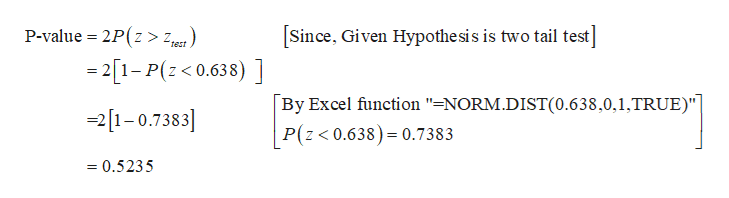# The drug Lipitor is meant to reduce cholesterol and LDL cholesterol. In clinical trials, 19 out of 863 patients taking 10 mg of Lipitor daily complained of flulike symptoms. Suppose that it is known that 1.9% patients taking competing drugs complain of flulike symptoms.a) ) Determine the P-value for this hypothesis test, and draw a normal curve with an area representing the P-value shaded.(e) Interpret the P-value.  (f) Based on the P-value, what is the conclusion of the hypothesis test? Assume an α = 0.05 level of significance.

Question

The drug Lipitor is meant to reduce cholesterol and LDL cholesterol. In clinical trials, 19 out of 863 patients taking 10 mg of Lipitor daily complained of flulike symptoms. Suppose that it is known that 1.9% patients taking competing drugs complain of flulike symptoms.

a) ) Determine the P-value for this hypothesis test, and draw a normal curve with an area representing the P-value shaded.

(e) Interpret the P-value.

(f) Based on the P-value, what is the conclusion of the hypothesis test? Assume an α = 0.05 level of significance.

check_circleExpert Solution
Step 1

(a)

The appropriate Null and Alternative Hypotheses are given below:

From the given information, the claim of the problem is testing whether 1.9% of the patients taking competing drugs complain of flulike symptoms or not.

Null hypothesis:

H0p = 0.019.

That is, the proportion of patients who takes competing drugs complain of flulike symptoms is 0.019.

Alternative hypothesis:

Ha≠0.019.

That is, the proportion of patients who takes competing drugs complain of flulike symptoms is not equal to 0.019.

Step 2

The test statistic value is ­0.638 and it is obtained below:

Here, the sample size is, n = 863; in those 19 taking 10 mg of Lipitor daily complained of flulike symptoms. The sample proportion is   =0.022(=19/863)

Step 3

The P-value is 0.5235 and it is calcula...help_outlineImage TranscriptioncloseSince, Given Hypothe sis is two tail test] P-value 2P(z > zt) -21-P(z0.638) ] By Excel function "=NORM.DIST(0.638,0,1,TRUE)"] P(z< 0.638) 0.7383 21-0.7383 =0.5235 fullscreen

### Want to see the full answer?

See Solution

#### Want to see this answer and more?

Solutions are written by subject experts who are available 24/7. Questions are typically answered within 1 hour*

See Solution
*Response times may vary by subject and question
Tagged in

### Statistics